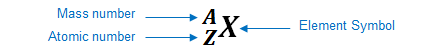# Problem: Fill in the gaps in the following table, assuming each column represents a neutral atom:Symbol79BrProtons2582Neutrons3064Electrons4886Mass no.222207Complete the fourth row of the table.

###### FREE Expert Solution

We’re being asked to fill the gap in the fourth row of the table which is to determine the number of electrons.

An element can be represented as:You can determine the identity of the element using its atomic number:

Atomic number (Z):

represents the identity of the element
reveals the number of protons
in a neutral element:

# of protons = # of electrons

Mass number (A)

reveals the number of protons and neutrons

mass number = # of protons + # of neutrons

94% (3 ratings)###### Problem Details
Fill in the gaps in the following table, assuming each column represents a neutral atom:

 Symbol 79Br Protons 25 82 Neutrons 30 64 Electrons 48 86 Mass no. 222 207

Complete the fourth row of the table.